Figure 11.2 Adding an imported raster image to a plot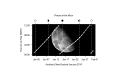R code
 Figure 11.3 Drawing an imported raster with grid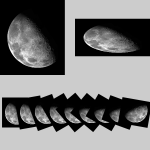R code
 Figure 11.4 Drawing an imported vector image with grid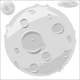R code
 Figure 11.5 Adding an imported vector image to a plot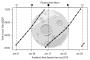R code
 Figure 11.6 Variations on the Moon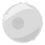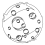R code
 Figure 11.7 Breaking a vector image into paths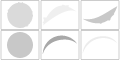R code
 Figure 11.8 A grImport2 rendering of the cartoon moon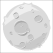R code
 Figure 11.9 A test image for importing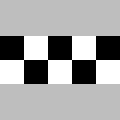R code
 Figure 11.10 The test image from grImportR code
 Figure 11.11 The test image from grImport2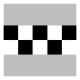R code
 Figure 11.12 An image with radial gradients on a normal device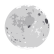R code
 Figure 11.13 An image with radial gradients on a gridSVG device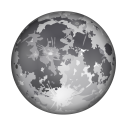R code
 Figure 11.14 Adding a complex imported vector image to a plot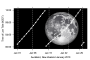R code